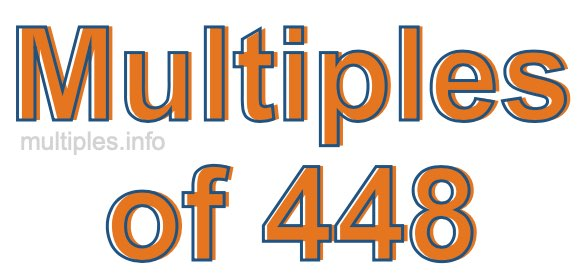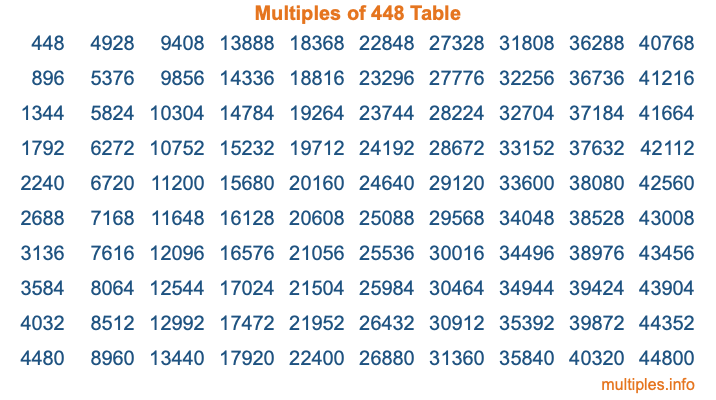Multiples of 448Welcome to the Multiples of 448 page. Here we will first teach you everything you will ever need to know about the multiples of 448, and then give you a study guide summary of everything we taught you to make sure you remember it all. Use this page to look up facts and learn information about the multiples of 448. This page will make you a multiples of four hundred forty-eight expert!

Definition of Multiples of 448
Multiples of 448 are all the numbers that when divided by 448 equal an integer. Each of the multiples of 448 are called a multiple. A multiple of 448 is created by multiplying 448 by an integer.

Therefore, to create a list of multiples of 448, you start with 1 multiplied by 448, then 2 multiplied by 448, then 3 multiplied by 448, and so on for as long as you want. Thus, the list of the first five multiples of 448 is 448, 896, 1344, 1792, and 2240. To see a larger list of multiples of 448, see the printable image of Multiples of 448 further down on this page. We also have a category where you can choose any nth multiple of 448.

Multiples of 448 Checker
The Multiples of 448 Checker below checks to see if any number of your choice is a multiple of 448. In other words, it checks to see if there is any number (integer) that when multiplied by 448 will equal your number. To do that, we divide your number by 448. If the the quotient is an integer, then your number is a multiple of 448.

Is  a multiple of 448?

Least Common Multiple of 448 and ...
A Least Common Multiple (LCM) is the lowest multiple that two or more numbers have in common. This is also called the smallest common multiple or lowest common multiple and is useful to know when you are adding our subtracting fractions. Enter one or more numbers below (448 is already entered) to find the LCM.

Check out our LCM Calculator if you need more details about the Least Common Multiple or if you need the LCM for different numbers for adding and subtraction fractions.

nth Multiple of 448
As we stated above, 448 is the first multiple of 448, 896 is the second multiple of 448, 1344 is the third multiple of 448, and so on. Enter a number below to find the nth multiple of 448.

th multiple of 448

Multiples of 448 vs Factors of 448
448 is a multiple of 448 and a factor of 448, but that is where the similarities end. All postive multiples of 448 are 448 or greater than 448. All positive factors of 448 are 448 or less than 448.

Below is the beginning list of multiples of 448 and the factors of 448 so you can compare:

Multiples of 448: 448, 896, 1344, 1792, 2240, etc.

Factors of 448: 1, 2, 4, 7, 8, 14, 16, 28, 32, 56, 64, 112, 224, 448

As you can see, the multiples of 448 are all the numbers that you can divide by 448 to get a whole number. The factors of 448, on the other hand, are all the whole numbers that you can multiply by another whole number to get 448.

It's also interesting to note that if a number (x) is a factor of 448, then 448 will also be a multiple of that number (x).

Multiples of 448 vs Divisors of 448
The divisors of 448 are all the integers that 448 can be divided by evenly. Below is a list of the divisors of 448.

Divisors of 448: 1, 2, 4, 7, 8, 14, 16, 28, 32, 56, 64, 112, 224, 448

The interesting thing to note here is that if you take any multiple of 448 and divide it by a divisor of 448, you will see that the quotient is an integer.

Multiples of 448 Table
Below is an image of the first 100 multiples of 448 in a table. The table is in chronological order, column by column. The first column has the first ten multiples of 448, the second column has the next ten multiples of 448, and so on.The Multiples of 448 Table is also referred to as the 448 Times Table or Times Table of 448. You are welcome to print out our table for your studies.

Negative Multiples of 448
Although not often discussed or needed in math, it is worth mentioning that you can make a list of negative multiples of 448 by multiplying 448 by -1, then by -2, then by -3, and so on, to get the following list of negative multiples of 448:

-448, -896, -1344, -1792, -2240, etc.

Multiples of 448 Summary
Below is a summary of important Multiples of 448 facts that we have discussed on this page. To retain the knowledge on this page, we recommend that you read through the summary and explain to yourself or a study partner why they hold true.

There are an infinite number of multiples of 448.

A multiple of 448 divided by 448 will equal a whole number.

448 divided by a factor of 448 equals a divisor of 448.

The nth multiple of 448 is n times 448.

The largest factor of 448 is equal to the first positive multiple of 448.

448 is a multiple of every factor of 448.

448 is a multiple of 448.

A multiple of 448 divided by a divisor of 448 equals an integer.

448 divided by a divisor of 448 equals a factor of 448.

Any integer times 448 will equal a multiple of 448.

Multiples of a Number
Here you can get the multiples of another number, all with the same attention to detail as we did for multiples of 448 on this page.

Multiples of
Multiples of 449
Did you find our page about multiples of four hundred forty-eight educational? Do you want more knowledge? Check out the multiples of the next number on our list!